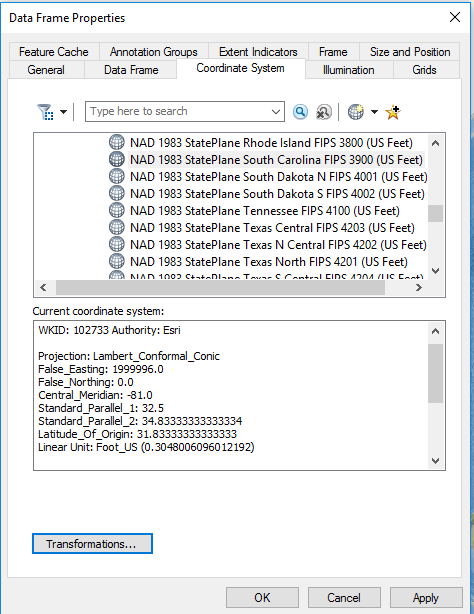673
3
05-29-2017 08:16 AMOccasional Contributor II

I am using ArcMap 10.4.1 reviewing some concepts of a projection.  The data frame properties look like the below screen shots.  Under the Standard Parallels is listed 2:34.83333 or 1:32.5.  are these standard parallels meant to be read as degrees and minutes ?

1 Solution

Accepted SolutionsbyMVP Esteemed Contributor

Standard Parallel 1 and Standard Parallel 2 are 32.5 degrees and 34.833333 degrees respectively. The units are in decimal degrees.

Think Location
3 RepliesOccasional Contributor IIbyMVP Legendary Contributor

The visual rules

45.50000   Decimal degrees

45 30.000  degrees decimal minutes

45 30 30.0000 degrees minutes seconds

The integer portions break down denote major D M or S

the decimal number indicates what is not integer.

So in the case of decimal degrees above, there is no integer, hence it is decimal degrees

In the case of degrees decimal minutes, the minutes has the decimal a the degrees portion is an integer

In the case of degrees, minutes, seconds, the seconds have decimals and the degrees and minutes portions are integers

Variants exist, just remember that decimal degrees is what is needed in the digital world and all other variants must be converted to them.  From there, you can introduce a projected coordinate system using the decimal degree data as inputsbyMVP Esteemed Contributor

Standard Parallel 1 and Standard Parallel 2 are 32.5 degrees and 34.833333 degrees respectively. The units are in decimal degrees.

Think Location